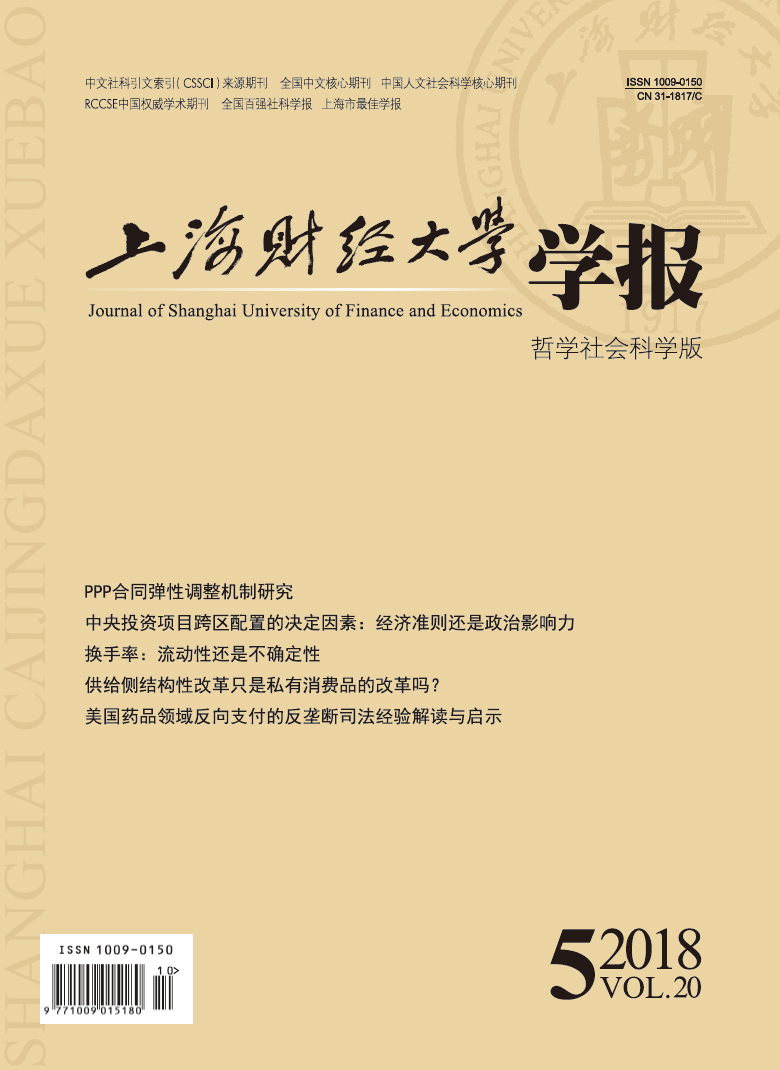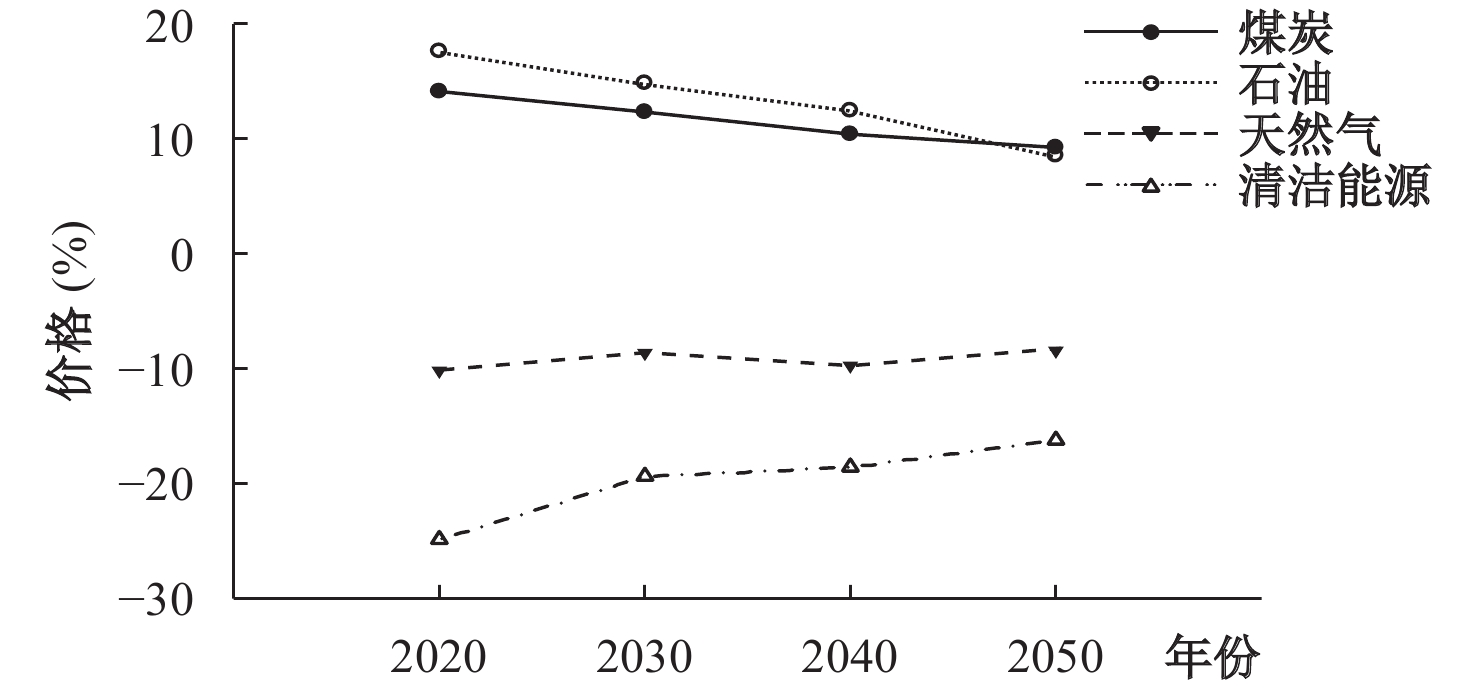﻿ 清洁能源补贴改革对产业发展和环境污染影响研究——基于动态CGE模型分析
 上海财经大学学报2018, Vol. 20Issue (5): 44-570

#### 文章信息上海财经大学2018年20卷第5期

Xu Xiaoliang

Impacts of the Clean Energy Subsidy Reform on Industry Development and Environment Pollution: An Analysis Based on a Dynamic CGE Model

Journal of Shanghai University of Finance and Economics, 2018, 20(5): 44-57.

### 文章历史《上海财经大学学报》
2018第20卷第5期

 时间 清洁能源政策名称 重点内容 2006 中华人民共和国可再生能源法 可再生能源发电站以并网时间为根据，通过排队纳入可再生能源补贴目录，获得国家下发的可再生能源发电补贴 2008 资源综合利用企业所得税优惠目录，资源综合利用产品及劳务增值税优惠目录 秸秆能源化补贴：对利用秸秆等生产电力、热力及燃气实行所得税、增值税优惠 2009 关于完善风力发电上网电价政策的通知 实行风电费用分摊制度 2013 关于发挥价格杠杆作用促进光伏产业健康发展的通知 明确分布式光伏发电项目的补贴标准为0.42元/千瓦（含税） 2014 能源发展战略行动计划（2014–2020） 实行差别化能源价格政策，加强能源需求侧管理，推行合同能源管理 2015 2015年农村沼气工程转型升级工作方案 投资补贴：对规模化大型沼气工程、规模化生物天然气试点工程予以投资补助 2016 生物质燃料锅炉补贴 对生物质锅炉进行资金补助 2016 关于太阳能热发电标杆上网电价政策的通知 核定太阳能热发电标杆上网电价为每千瓦时1.15元，对太阳能热发电企业采取税费减免、财政补贴等措施 2016 国家能源局关于做好“三北”地区可再生能源消纳工作的通知 按新能源标杆上网电价与当地煤电标杆上网电价的差额享受可再生能源补贴 2017 关于开展中央财政支持北方地区冬季清洁取暖试点工作的通知 支持试点城市推进清洁方式取暖替代散煤燃烧取暖

 ${Q_T} = \sum {(TVA + IP) = } \sum {(TVA + } {A_{ip}}(\pi _1^{1/\sigma }IP_1^{\sigma - 1/\sigma } + \pi _2^{1/\sigma }IP_2^{\sigma - 1/\sigma }))$ (1)

 ${P_E} = {\kappa _1}\sum {{P_1} + \left( {1 - {\kappa _1}} \right)\sum {{P_2}} }$ (2)

 $\frac{{{P_1}}}{{{P_2}}} = {\left(\frac{{I{P_1}}}{{I{P_2}}}\right)^{ - \frac{1}{\sigma }}}$ (3)

 $\max{P_E}{Q_T} = \max\left({\kappa _1}\sum {{P_1} + \left( {1 - {\kappa _1}} \right)\sum {{P_2}} } \right)\left(\sum {\Big(TVA + } {A_{ip}}\Big(\pi _1^{1/\sigma }IP_1^{\sigma - 1/\sigma } + \pi _2^{1/\sigma }IP_2^{\sigma - 1/\sigma }\Big)\Big)\right)$ (4)

 $S = {\left( {\frac{{\alpha {P_E}}}{{{P_1}}}} \right)^{\frac{1}{{1 - \alpha }}}}A{Q_E}$ (5)

 $\max{P_E}{Q_T} = \max\biggr({\kappa _1}\sum {\left( {{P_1} + B} \right) + \left( {1 - {\kappa _1}} \right)\sum {{P_2}} } )(\sum {(TVA + } {A_{ip}}(\pi _1^{1/\sigma }IP_1^{\sigma - 1/\sigma } + \pi _2^{1/\sigma }IP_2^{\sigma - 1/\sigma }))\biggr)$ (6)

 $B = {P_1}/{\alpha _1}$ (7)

 $S = {\left( {\frac{{{P_E}}}{B}\frac{\alpha }{{{\alpha _1}}}} \right)^{\frac{1}{{1 - \alpha }}}}A{Q_E}$ (8)

 ${\left( {\frac{{{{\rm{P}}_{\rm{E}}}}}{B}\frac{\alpha }{{{\alpha _{\rm{1}}}}}} \right)^{\frac{1}{{1 - \alpha }}}}A{Q_E}{\rm{ = }}\sum {(TVA + } {A_{ip}}(\pi _1^{1/\sigma }IP_1^{\sigma - 1/\sigma } + \pi _2^{1/\sigma }IP_2^{\sigma - 1/\sigma }))$ (9)

 $B = {P_E}\frac{\alpha }{{{\alpha _1}}}{\Biggr( {\frac{{\sum {(TVA + } {A_{ip}}(\pi _1^{1/\sigma }IP_1^{\sigma - 1/\sigma } + \pi _2^{1/\sigma }IP_2^{\sigma - 1/\sigma }))}}{{A{Q_E}}}} \Biggr)^{\frac{1}{{1 - \alpha }}}} = {P_E}\frac{\alpha }{{{\alpha _1}}}{\left( {\frac{{{Q_T}}}{{A{Q_E}}}} \right)^{\frac{1}{{1 - \alpha }}}}$ (10)

 比较静态分析 清洁能源补贴政策对相关变量的影响 价格A ${\displaystyle\frac{{\partial B}}{{\partial {P_E}}}}$ 正向关系 总产出 ${\displaystyle\frac{{\partial B}}{{\partial {Q_T}}}}$ 正向关系 总体技术水平 ${\displaystyle\frac{{\partial {B}}}{{\partial {A}}}}$ 负向关系图 1 动态CGE模型的模块结构和基本框架

 ${Q_T} = AL_t^\gamma E_t^\eta K_t^{1 - \gamma - \eta } = \sum {(TVA + IP} )$ (11)

 $TVA = A\Big(\alpha _1^{1/\kappa }{L^{(\kappa - 1)/\kappa }} + \alpha _2^{1/\kappa }{K^{(\kappa - 1)/\kappa }} + \alpha _3^{1/\kappa }{E^{(\kappa - 1)/\kappa }}\Big)$ (12)

 $S = \Biggr(\sum {{G_{_E}} + \sum\limits_{n = 1}^{42} {F{x_n}} } + \sum {{C_E}} \Biggr){P_E}$ (13)

 ${G_{_E}} = (1 - {R_{GS}})GDP - B$ (14)

 $F{x_n} = Y - w - rK - T + B$ (15)

 ${C_E} = w + B - {C_i}$ (16)

 ${P_E} = \biggr(\sum {PQ + \sum {PV} } \biggr)\operatorname{int} a$ (17)

 ${P_o} = {P_E} + PR + PW + T + B$ (18)

 $E = {A_e}({\lambda _1}{Q_C} \times 0.714\;3 + {\lambda _2}{Q_o} \times 1.428\;6 + {\lambda _3}{Q_G} \times 12.143 + {\lambda _4}{Q_{CE}} \times 0.122\;9)$ (19)

 $IP = {A_{ip}}\Big(\pi _1^{1/\sigma }IP_1^{(\sigma - 1)/\sigma } + \pi _2^{1/\sigma }IP_2^{(\sigma - 1)/\sigma }\Big)$ (20)

 \begin{aligned}YE =& P{E_{TAX}} - P{E_{COST}}\;s.t.\;P{E_{TAX}} \\=& \sum {P{E_{P1}}Q{E_{Q1}}} + \sum {P{E_{P2}}Q{E_{Q2}}}P{E_{COST}} \\=& \sum {(P{E_{P2}} + Q{E_{Q2}}} )P{E_1}P{E_{AC}} \end{aligned} (21-23)

(二) 基础数据、变量选择和关键参数的确定

CGE模型中生产函数的弹性参数主要由经典统计方法、Bayesian方法和GME方法求得；需求函数是由居民效用函数推导而得，效用函数的参数常用经典计量经济方法估计。各要素之间的替代系数,需求函数中的弹性系数等关键参数值的选取主要借鉴计量经济学方法和其他CGE模型的相关文献确定，其他参数通过对社会核算矩阵进行校准（Calibration）的方法确定。

(三) 模型动态化处理过程

 $Q(E) = \theta {P_E}\biggr(\sum {(Q{{(E)}_{T - 1}} + {B_{T - 1}}\biggr) + \sum {Q{{(E)}_T}} } )$ (24)

 时间 能源生产弹性系数 能源消费弹性系数 能源消费总量 煤炭消费总量 石油消费总量 天然气消费总量 清洁能源消费总量 2012 0.41 0.51 402 138 240 914 68 006 18 810 34 003 2011 0.95 0.77 387 043 238 033 64 728 17 400 27 840 2010 0.86 0.69 360 648 220 959 61 738 14 297 27 945 2009 0.34 0.53 336 126 215 879 54 890 11 959 23 918 2008 0.52 0.31 320 611 204 888 53 335 10 784 22 442 2007 0.56 0.61 311 442 199 441 52 736 9 257 19 075 2006 0.54 0.76 286 467 183 919 49 924 7 502 17 331 2005 0.98 1.19 261 369 167 086 46 727 6 136 16 048 2004 1.54 1.67 230 281 148 352 45 466 5 336 14 302 2003 1.41 1.62 197 083 128 287 38 964 4 595 11 946 2002 0.66 0.99 169 577 108 413 35 553 3 826 11 638 2001 0.77 0.7 155 547 102 727 32 789 3 610 11 280 2000 0.6 0.54 146 964 100 707 32 308 3 202 9 314 均速 − − 14.47% 11.60% 9.21% 40.63% 22.09%

 关键指标 2007年 2008年 2009年 2010年 2011年 2012年 人均能源供给量 1.14 1.11 1.18 1.14 1.23 1.29 人均能源需求量 2.27 2.25 2.26 2.12 2.05 2.12 人均能源赤字/盈余 –1.13 –1.14 –1.08 –0.98 –0.82 –0.83 人均能源压力系数 1.73 1.68 1.65 1.57 1.49 1.47 单位GDP能耗 0.560 0.544 0.529 0.513 0.472 0.455

 时间 废气排放总量（万吨） SO2（万吨） 工业废水（亿吨） 工业固体废物排放（万吨） 污染物治理投入（亿元） 2000 138 145 1 995 415 81 608 1 010 2001 160 863 1 948 433 88 840 1 107 2002 175 257 1 927 439 94 509 1 367 2003 198 906 2 159 459 100 428 1 628 2004 237 696 2 255 482 120 030 1 910 2005 268 988 2 549 526 134 449 2 388 2006 330 990 2 589 537 151 541 2 566 2007 388 169 2 468 557 175 632 3 387 2008 403 866 2 321 572 190 127 4 937 2009 − 2 214 589 203 943 5 258 2010 − 2 185 617 240 944 7 612 2011 − 2 218 659 322 772 7 114 2012 − 2 118 684 329 044 8 253 均速 32.45% 8.17% 12.68% 31.02% 62.86%

 补贴改革场景 补贴强度 补贴标准 场景一（P1） 国家基本补贴 国家0.42元每千瓦时 场景二（P2） 国家基本补贴+弱地方补贴 国家0.42+地方0.1元每千瓦时 场景三（P3） 国家基本补贴+强地方补贴 国家0.42+地方0.2元每千瓦时

(二) 清洁能源补贴改革的环境效应影响分析

1. 对能源价格和能源替代弹性的影响图 2 国家基本补贴改革场景一（P1）对能源价格的影响

 能源替代弹性 场景一（P1） 场景二（P2） 场景三（P3） 煤炭—石油 0.26 0.29 0.27 煤炭—天然气 1.34 1.47 1.44 煤炭—清洁能源 0.18 0.27 0.35 石油—天然气 0.49 0.52 0.51 石油—清洁能源 1.15 1.31 1.43 天然气—清洁能源 1.23 1.38 1.52

 比重 场景一（P1） 场景二（P2） 场景三（P3） 煤炭 62% 61% 61% 石油 15% 14% 12% 天然气 9% 15% 10% 清洁能源 14% 15% 17%

2. 对产业产出和能源消费的影响

 时间 政策场景 2020年 2030年 2040年 2050年 总产出 场景一（P1） 11.27 15.46 17.15 21.38 场景二（P2） 13.16 16.29 19.43 22.62 场景三（P3） 14.43 17.86 21.26 24.54

 政策场景 农业 工业 能源 环保 服务 建筑 运输 场景一（P1） 1.72 8.22 12.35 4.22 2.52 3.22 7.13 场景二（P2） 2.95 12.48 18.47 6.25 4.84 4.17 9.64 场景三（P3） 3.11 15.45 24.22 7.21 6.52 5.31 11.18

 政策场景 相关产业 能源消费 煤炭消费 石油消费 清洁能源消费 场景一（P1） 农业产业 1.68 –4.32 2.69 5.41 开采产业 10.18 5.69 8.86 16.62 工业产业 16.53 12.17 14.35 22.16 能源产业 14.16 7.52 10.36 18.67 建筑产业 6.58 3.62 8.59 10.43 交通产业 14.56 10.22 16.55 19.19 服务产业 7.49 4.66 9.32 11.43 环境保护 4.56 2.79 3.17 14.45 场景二（P2） 农业产业 1.97 –4.67 2.89 7.78 开采产业 10.75 5.24 8.26 18.59 工业产业 16.18 11.67 13.64 25.34 能源产业 14.02 7.26 10.12 19.61 建筑产业 6.52 3.35 7.84 12.47 交通产业 14.42 9.76 15.48 20.65 服务产业 7.23 4.16 9.08 13.12 环境保护 4.21 2.56 2.97 15.62 场景三（P3） 农业产业 1.93 –4.23 2.77 8.43 开采产业 10.58 5.12 8.03 20.29 工业产业 16.34 11.05 12.89 27.69 能源产业 14.12 7.08 10.34 20.88 建筑产业 6.41 3.26 7.45 14.09 交通产业 14.26 9.28 15.07 21.06 服务产业 7.04 4.02 8.59 14.35 环境保护 4.18 2.34 2.27 16.32

3. 能源系统和污染治理的影响

 清洁能源补贴 人均能源盈余/赤字 人均能源压力系数 单位GDP能耗 场景一（P1） –0.86 1.67 0.449 场景二（P2） –0.83 1.62 0.426 场景三（P3） –0.79 1.58 0.417

 指标 场景一（P1） 场景二（P2） 场景三（P3） 2020 2030 2040 2050 2020 2030 2040 2050 2020 2030 2040 2050 废水排放 2.45 2.29 1.98 1.76 2.37 2.16 1.88 1.65 2.29 2.01 1.82 1.56 废气排放 2.85 2.62 2.34 2.13 2.73 2.53 2.31 2.08 2.65 2.48 2.27 1.98 工业固体废物排放 4.27 3.96 3.73 3.55 4.18 3.84 3.61 3.47 4.09 3.77 3.52 3.39

 废水 废气 工业固体废物 场景一 场景二 场景三 场景一 场景二 场景三 场景一 场景二 场景三 农业产业 1.22 1.12 1.06 1.65 1.42 1.28 3.45 3.36 3.27 开采产业 2.24 2.09 1.89 2.62 2.56 2.41 4.62 4.34 4.15 工业产业 3.05 2.85 2.66 3.32 3.06 2.78 5.59 5.25 4.89 能源产业 2.11 1.98 1.77 2.87 2.46 2.31 4.38 4.09 3.78 建筑产业 1.36 1.21 1.06 2.09 1.88 1.67 3.23 3.03 2.82 交通产业 1.13 1.03 0.89 4.06 3.48 3.27 3.49 3.26 3.09 服务产业 1.79 1.56 1.37 2.65 2.45 2.32 3.66 3.18 2.72 环境保护 1.23 1.16 1.05 1.37 1.18 1.04 1.42 1.23 1.04图 3 不同政策场景下碳排放的变化情况

  郭菊娥, 钱冬, 吕振东, 等. 煤炭资源税调整测算模型及其效应研究[J].中国人口•资源与环境,2011(1).  景维民, 张璐. 环境管制、对外开放与中国工业的绿色技术进步[J].经济研究,2014(9).  李波. 煤炭资源税改革目标实现的困境与对策[J].中国人口•资源与环境,2013(1).  李钢, 董敏杰, 沈可挺. 强化环境管制政策对中国经济的影响——基于CGE模型的评估[J].中国工业经济,2012(11).  李虹, 熊振兴. 生态占用、绿色发展与环境税改革[J].经济研究,2017(7).  林伯强, 刘希颖, 邹楚沅, 等. 资源税改革: 以煤炭为例的资源经济学分析[J].中国社会科学,2012(2).  刘亦文, 胡宗义. 能源技术变动对中国经济和能源环境的影响——基于一个动态可计算一般均衡模型的分析[J].中国软科学,2014(4).  牛玉静, 陈文颖, 吴宗鑫. 全球多区域CGE模型的构建及碳泄漏问题模拟分析[J].数量经济技术经济研究,2012(11).  涂正革. 中国的碳减排路径与战略选择——基于八大行业部门碳排放量的指数分解分析[J].中国社会科学,2012(3).  魏巍贤. 基于CGE模型的中国能源环境政策分析[J].统计研究,2009(7).  姚昕, 蒋竺均, 刘江华. 改革化石能源补贴可以支持清洁能源发展[J].金融研究,2011(3).  张国兴, 高秀林, 汪应洛, 等. 政策协同: 节能减排政策研究的新视角[J].系统工程理论与实践,2014(3).  庄贵阳. 气候变暖与诺亚方舟[N]. 人民日报, 2006-04-05（03）.  Acemoglu D, Aghion P, Bursztyn L, et al. The environment and directed technical change[J].American Economic Review,2012,102(1):131–166.  Bella G, Massidda C, Mattana P. The relationship among CO2 emissions, electricity power consumption and GDP in OECD countries [J].Journal of Policy Modeling,2014,36(6):970–985.  Kawase R, Matsuoka Y, Fujino J. Decomposition analysis of CO2 emission in long-term climate stabilization scenarios [J].Energy Policy,2006,34(15):2113–2122.  Rader N A, Norgaard R B. Efficiency and sustainability in restructured electricity markets: The renewables portfolio standard[J].The Electricity Journal,1996,9(6):37–49.  Wiser R, Bolinger M, Barbose G. Using the federal production tax credit to build a durable market for wind power in the United States[J].The Electricity Journal,2007,20(9):77–88.
Impacts of the Clean Energy Subsidy Reform on Industry Development and Environment Pollution: An Analysis Based on a Dynamic CGE Model
Xu Xiaoliang
School of Economics and Management, Nanjing University of Science and Technology, Jiangsu Nanjing 210094, China
Summary: Clean energy subsidies are important policy tools to promote the development of related industries and achieve resource and environmental goals in China. In view of a series of serious environmental pollution problems caused by fossil energy consumption, the call for increasing the clean energy use and improving the utilization of clean energy to improve and optimize the energy consumption structure has become more and more intense in recent years. However, previous studies have focused on the energy price reform and the impact of the subsidy scale on the social economy, focusing on the analysis of the process and effect of energy subsidy policies on macroeconomic operations while lacking quantitative research on the impact of relevant industries and environmental systems. In the clean energy subsidy program, different subsidy policy schemes are quite different. How much influence does the clean energy subsidy reform design have on different industry development and environmental pollution control? It is worth further research. The paper constructs a dynamic computable general equilibrium model （DCGE） to appraise the impact of clean energy subsidies on industry development and environmental governance based on a " national + local” scenario from a long-term latitude. The results show that, overall speaking, clean energy subsidies are helpful to promote the energy price reform. Due to the price advantage brought by clean energy subsidy policies, elasticity of substitution between fossil energy and clean energy can be changed greatly, which will increase the total output of related industries, improve the energy consumption structure, and reduce the emission intensity of major pollutants. However, the impacts of different subsidy programs greatly differ from each other. National basic subsidy policies can improve the competitiveness of clean energy to some extent, and strong local subsidy policies can be more effective to promote the development of clean energy industries, improve energy consumption per unit GDP and per capita energy surplus, reduce the environmental damage, and optimize the energy consumption structure. Therefore, the use of the " national + strong local” design is more conducive to clean energy industry development and environmental governance. The main contributions of this paper are to introduce factors such as clean energy industry and resource environment into the subsidy reform research, effectively assess the differences between different programs on industrial development and environmental systems, and study the subsidy intensity of different topics such as national and local governments in subsidy policies. The impact of alternative elasticity, industrial development and environmental pollution control makes clean energy subsidy policies more in line with the reality of social development. The results of this paper can improve the feasibility of the clean energy subsidy program, and provide reference for the formulation and sustainable development of clean energy subsidy policies related to the national economy and the people’s livelihood.
Key words: clean energy subsidy reform     dynamic DCGE model     industry development